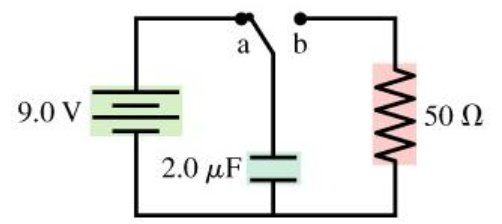# Problem: The switch in the figure has been in position a for a long time. It is changed to position b at t = 0 s.A. What is the charge Q on the capacitor at t = 50 µs?B. What is the current I through the resistor at t = 50 µs?

###### FREE Expert Solution

Charge decay formula is:

$\overline{){\mathbf{Q}}{\mathbf{\left(}}{\mathbf{t}}{\mathbf{\right)}}{\mathbf{=}}{\mathbf{C}}{{\mathbf{V}}}_{{\mathbf{0}}}{\mathbf{\left(}}{{\mathbf{e}}}^{\frac{\mathbf{-}\mathbf{t}}{\mathbf{\tau }}}{\mathbf{\right)}}}$

Expression of voltage across the capacitor is:

$\overline{){{\mathbf{V}}}_{{\mathbf{C}}}{\mathbf{\left(}}{\mathbf{t}}{\mathbf{\right)}}{\mathbf{=}}{{\mathbf{V}}}_{{\mathbf{0}}}{{\mathbf{e}}}^{\frac{\mathbf{-}\mathbf{t}}{\mathbf{R}\mathbf{C}}}}$

This is because the time constant τ is given as, τ = RC

95% (17 ratings)###### Problem DetailsThe switch in the figure has been in position a for a long time. It is changed to position b at t = 0 s.

A. What is the charge Q on the capacitor at t = 50 µs?

B. What is the current I through the resistor at t = 50 µs?